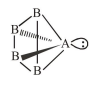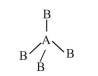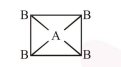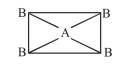# If AB4 molecule is a polar molecule,

Question:

If $\mathrm{AB}_{4}$ molecule is a polar molecule, a possible geometry of $\mathrm{AB}_{4}$ is :

1. Square pyramidal

2. Tetrahedral

3. Square planar

4. Rectangular planar

Correct Option: 1

Solution:

(1) If $\mathrm{AB}_{4}$ molecule is a square pyramidal then it has one lone pair and their structure should beand it should be polar because dipole moment of lone pair of 'A' never be cancelled by others.

(2) If $\mathrm{AB}_{4}$ molecule is a tetrahedral then it has no lone pair and their structure should beand it should be non polar due to perfect symmetry.

(3) If $\mathrm{AB}_{4}$ molecule is a square planar thenit should be non polar because vector sum of dipole moment is zero.

(4) If $\mathrm{AB}_{4}$ molecule is a rectangular planar thenit should be non polar because vector sum of dipole moment is zero.